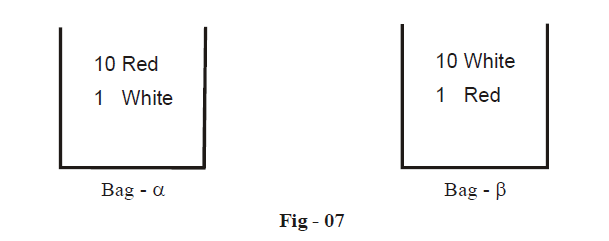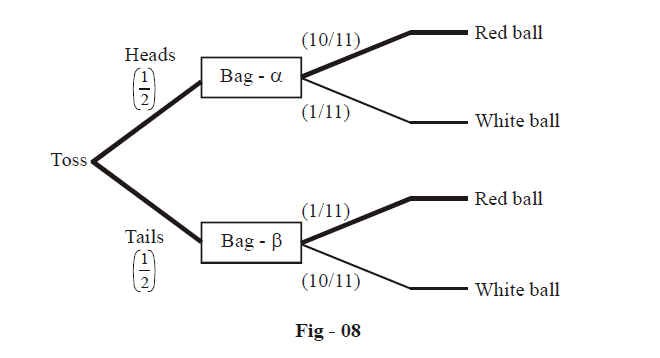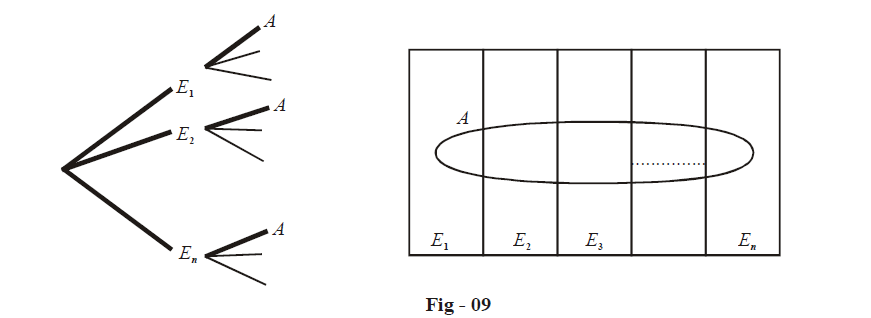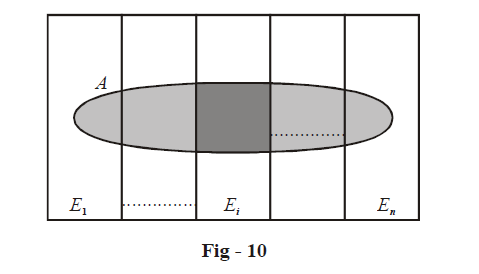# Inverse Probability and Bayes Theorem

Go back to  'Probability'

Learn from the best math teachers and top your exams

• Live one on one classroom and doubt clearing
• Practice worksheets in and after class for conceptual clarity
• Personalized curriculum to keep up with school

To understand the underlying concept of inverse probability, lets start with a very simple example.

Suppose that you have two bags $$\alpha \,and\,\beta$$ , one containing 10 red and 1 white balls, and the second containing 10 white and 1 red balls.You play a game with your friend. The friend tosses a fair coin, without telling you the outcome and if he gets a Head he withdraws a ball from Bag- $$\alpha$$  while if he gets a Tail, he withdraws a ball from Bag– $$\beta$$, with you looking away all the time. After doing this once, he has a red ball in his hand. Which bag do think is the more likely one from which this ball was drawn? Intuition immediately tells us that it should be Bag– $$\alpha$$, since it has a large number of red balls. What we need to do now is quantify the inverse probability of the ball being drawn from Bag– $$\alpha$$ and Bag– $$\beta$$, the word ‘inverse’ being used since you are trying to find the probability of an event that has already taken place, using information from a subsequent event. We do this intuitively all the time: “India’s scorecard against Pakistan in yesterday’s match had a century.” “Oh, it would most likely have been Tendulkar!” So here, the speaker is expressing his conviction that the century-scorer must have been Tendulkar, since he has in his mind the information about the various players, and he thinks Tendulkar is the best.

Coming back to our bags and balls, let us draw a tree diagram highlighting the various possible actions your friend can take (the brackets show the probabilities of the corresponding paths)Now comes the crucial part. Note that the total probability of selecting a red ball is the sum of the probabilities of the two darkened paths (one through Bag– $$\alpha$$, one through Bag–$$\beta$$). This is

$P\left( \text{Red}\,\text{Ball} \right)=\frac{1}{2}\times \frac{10}{11}+\frac{1}{2}\times \left( \frac{1}{11} \right)=\frac{1}{2}$

Now, the probability of selecting a red ball through Bag –$$\alpha$$  corresponds to the upper path only, and it equals

$P\left( \text{Red}\,\text{Ball from Bag-}\alpha \right)=\frac{1}{2}\times \frac{10}{11}=\frac{5}{11}$

Similarly,

$P\left( \text{Red}\,\text{Ball from Bag-}\beta \right)=\frac{1}{2}\times \frac{1}{11}=\frac{1}{22}$

Finally, it should now be intuitively obvious that

\begin{align}&\qquad\; P\left\{ \begin{gathered} \text{Bag selected was Bag-}\alpha \text{ } \\ \text{given that ball is red} \\ \end{gathered} \right\}=\frac{P\left( \text{Red ball from Bag-}\alpha \right)}{P\left( \text{Red ball} \right)} \\ &\qquad\qquad\qquad\qquad\qquad\qquad \qquad\quad\;\;=\frac{5/22}{1/2} \\ & \qquad\qquad\qquad\qquad\qquad\qquad \qquad\quad\;\;=\frac{10}{11} \\ & \text{while}\,\,P\left\{ \begin{gathered} \text{Bag selected was Bag-}\beta \text{ } \\ \text{given that ball is red} \\ \end{gathered} \right\}=\frac{P\left( \text{Red ball from Bag-}\beta \right)}{P\left( \text{Red ball} \right)} \\ & \qquad\qquad\qquad\qquad\qquad\qquad \qquad\quad\;\;=\frac{1/22}{1/2} \\ &\qquad\qquad\qquad\qquad\qquad\qquad \qquad\quad\;\;=\frac{1}{11} \\ \end{align}

Note what a huge difference there is between the two probabilities, which was expected. Also expected is the fact that the two probabilities sum to 1.

This, then, is the essence of calculating inverse probabilities. We are given the information that an event E has occurred. This event E can occur through n paths Paths1, Path2, ....., Pathn. We want to find the probability that E occurred through some particular path, say Pathi, which is

$P\left( E\,\text{occurred through Pat}{{\text{h}}_{i}} \right)=\frac{P\left( \text{Pat}{{\text{h}}_{i}} \right)}{P\left( \text{Pat}{{\text{h}}_{1}} \right)+P\left( \text{Pat}{{\text{h}}_{2}} \right)+...+P\left( \text{Pat}{{\text{h}}_{n}} \right)}$

Let us write this in standard terminology, which will give us the Baye’s theorem.

Suppose that the sample space consists of n mutually exclusive events E1, E2 ..., En. Now, an event A occurs, which could have resulted from any of the events Ei (For example, think of A as obtaining a red ball in the previous example, while E1 and E2 are selecting Bag-$$\alpha$$ and Bat-$$\beta$$ respectively). We intend to find P(Ei /A), i.e., the probability that Ei occurred given that A has occurred. There are now two ways to do the visualisation:The left hand tree we have already explained. The right hand side shows that A is an event that has occurred, which must have been a result of one of the Ei occurring (i.e. one of the Ei’s must occur for A to occur)

From the tree, evaluating P(Ei /A) has already been explained:

\begin{align}& P\left( {{E}_{i}}/A \right)=\frac{P\left( \text{Path}\,\,\text{to}\,A\,\,\text{through}\,\,{{E}_{i}} \right)}{\sum\limits_{j=1}^{n}{P\left( \text{Path}\,\,\text{to}\,A\,\,\text{through}\,\,{{E}_{j}} \right)}} \\ &\qquad\qquad =\frac{P\left( {{E}_{i}}\,\text{occurs and }\,\mathbf{then}\,\,A\,\,\text{occurs} \right)}{\sum\limits_{j=1}^{n}{P\left( {{E}_{j}}\,\,\text{occurs}\,\,\text{and}\,\,\text{then}\,\,A\,\,\text{occurs} \right)}} \\ & \qquad\qquad=\frac{P\left( {{E}_{i}}\,\text{occurs} \right)\times P\left\{ A\,\,\text{occurs given that }{{E}_{i}}\,\text{has occured} \right\}}{\sum\limits_{j=1}^{n}{P\left( {{E}_{j}}\,\text{occurs} \right)\times P\left\{ A\,\,\text{occurs given that }{{E}_{j}}\,\text{has occured} \right\}}} \\ &\qquad\qquad =\frac{P\left( {{E}_{i}} \right)P\left( A/{{E}_{i}} \right)}{\sum\limits_{j=1}^{n}{P\left( {{E}_{j}} \right)P\left\{ A\,/\,{{E}_{j}} \right\}}} \\ \end{align}

The same relation follows from the second figure:\begin{align}& P\left( {{E}_{i}}/A \right)=\frac{P\left( \text{Darkly shaded region} \right)}{P\left( \text{Total shaded region} \right)} \\\\ &\qquad\qquad =\frac{P\left( \text{A}\cap {{E}_{i}} \right)}{\sum\limits_{j\text{ =}\,\text{1}}^{n}{P\left( A\cap {{E}_{j}} \right)}} \\\\ &\qquad\qquad =\frac{P\left( {{E}_{i}} \right)P\left( A/{{E}_{i}} \right)}{\sum\limits_{j\text{ =}\,\text{1}}^{n}{P\left( {{E}_{j}} \right)P\left( A/{{E}_{j}} \right)}} \\ \end{align}

Thus, the famous Baye’s theorem is

$\boxed{\,P\left( {{E_i}/A} \right) = \frac{{P\left( {{E_i}} \right)P\left( {A/{E_i}} \right)}}{{\sum\limits_{j = 1}^n {P\left( {{E_j}} \right)P\left( {A/{E_j}} \right)} }}\,}$

The name “inverse” stems from the fact that this relation gives us P(Ei / A) in terms of P(A/Ej). The theorem is also known as a theorem on the probability of causes. The reason should be obvious.

In the examples following this, we’ll be using the tree diagram to calculate inverse probabilities. With sufficient practice, you’ll eventually not require drawing the tree diagram because by then you’ll be quite comfortable in using Baye’s theorem directly.

Probability
grade 11 | Questions Set 1
Probability
Probability
grade 11 | Questions Set 2
Probability